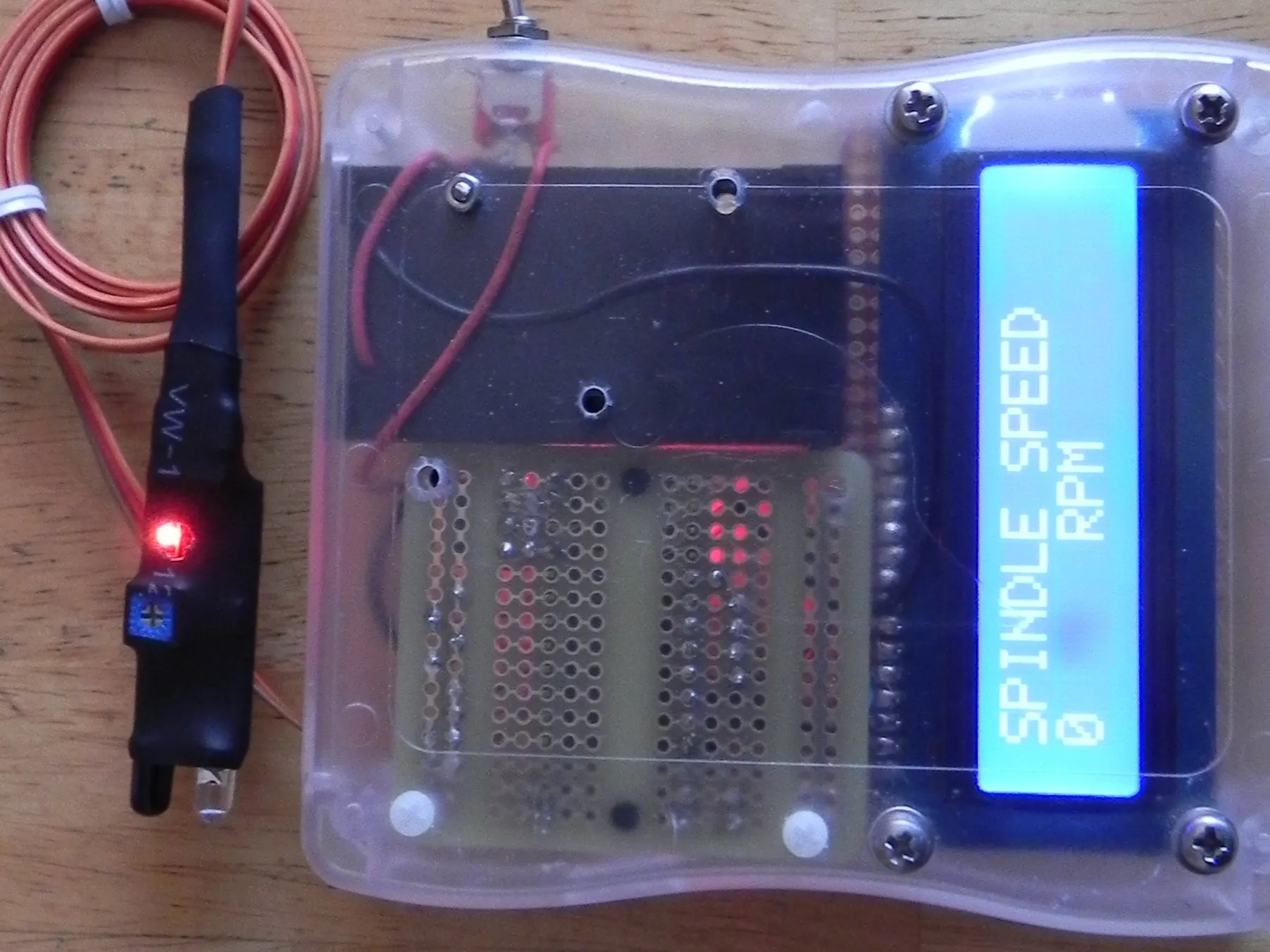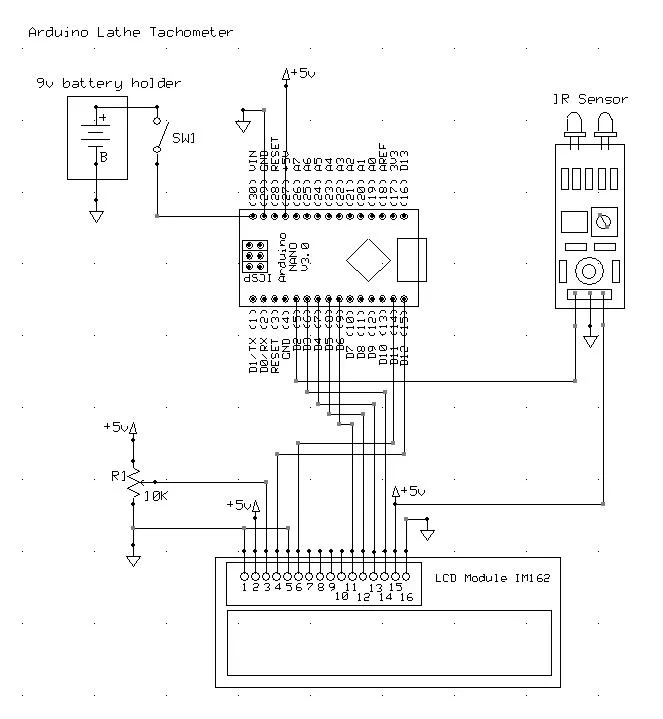# Arduino IR Lathe Tachometer

Build a tachometer for your metal lathe using an Arduino Nano, a two line LCD display, and an IR sensor.

IntermediateFull instructions provided6 hours6,475## Schematics

### Arduino Lathe Tachometer## Code

### Arduino code for Lathe Tachometer

Arduino
This program was used at one time with a reed relay, for a bicycle cadence counter I was building, but it works all right with the input from the IR sensor. If I need to reuse it, all variables are still in place. You can remove all the references to the cadence counter if you wish.
```// Tachometer_For_Lathe_IR
// Display RPM rate of lathe spindle

// Uses:
// Arduino Nano V 3.0
// OSOYOO IR Infrared Sensor (available on Amazon)
// IM162 LCD Display (similar ones available on Amazon)

// Include the LCD and Timer library code:
#include <LiquidCrystal.h>
#include <TimerOne.h>

// Initialize the library by associating any needed LCD interface pin
// with the arduino pin number it is connected to
const int rs = 12, en = 11, d4 = 6, d5 = 5, d6 = 4, d7 = 3;
LiquidCrystal lcd(rs, en, d4, d5, d6, d7);

// RPM values to track
const int IRSensorPin = 2;  // the number of the IR sensor input pin
const int ledPin = 13;          // the number of the LED pin on Nano
int ledState = HIGH;           // the current state of the output pin

// Note: This program was used at one time with a reed relay, for a bicycle cadence counter,
//       but it works all right with the input from the IR sensor.
//       If I need to reuse it, all variables are still in place.
int inputState;                          // the current state from the input pin
int lastInputState = LOW;        // the previous InputState from the input pin
long lastDebounceTime = 0;   // the last time the output pin was toggled
long debounceDelay = 5;        // the debounce time; increase if the output flickers
long time;
long endTime;
long startTime;
int RPM = 0;
//int lnCount = 0;        // counter for averaging RPM
//int avgRPM = 0;      // averaging total

//long voltage = 0;           // Value read from the pot
//long outputValue = 0;   // Value output to the PWM (analog out)
//int speedVal = 0;
//long rpm50 = 833333;
//volatile unsigned long cadTime = 0;  // use volatile for shared variables
//long timeC, endTimeC, startTimeC;
//int RPMC = 0;
float lnTime = 0;
//int speakerPin = 10;
//int lowRate;
//int hiRate;

// ---------------------------------------------------------------
void setup(void) {
pinMode(IRSensorPin, INPUT);
pinMode(ledPin, OUTPUT);
digitalWrite(ledPin, ledState);

Serial.begin(9600);
lcd.begin(16, 2); // Initialize LCD

// Startup text on display
lcd.clear();
lcd.print("LATHE IR.");
lcd.setCursor(0, 1);
lcd.print("TACHOMETER");
delay(2000);

endTime = 0;
Timer1.initialize(1000000);  // Set the timer to 60 rpm, 1,000,000 microseconds (1 second)
Timer1.attachInterrupt(timerIsr);  // Attach the service routine here

}

// ---------------------------------------------------------------
void loop(void) {
time = millis();

if (currentSwitchState != lastInputState) {
lastDebounceTime = millis();
}

if ((millis() - lastDebounceTime) > debounceDelay) {
if (currentSwitchState != inputState) {
inputState = currentSwitchState;
if (inputState == LOW) {
digitalWrite(ledPin, LOW);
calculateRPM(); // Real RPM from sensor
ledState = !ledState;
}
else {
digitalWrite(ledPin, HIGH);
}
}
}
lastInputState = currentSwitchState;
}

// ---------------------------------------------------------------
void calculateRPM() {
startTime = lastDebounceTime;
lnTime = startTime - endTime;
RPM = 60000 / (startTime - endTime);
endTime = startTime;
}

// --------------------------
// Custom ISR Timer Routine
// Timer set to rpm, see above
// --------------------------
void timerIsr()
{
// Print RPM every second
// RPM based on timer
Serial.println("---------------");
time = millis() / 1000;
Serial.print(time);
Serial.print(" RPM: ");
Serial.println(RPM);

lcd.clear();
lcd.print("SPINDLE SPEED");
lcd.setCursor(0, 1);
lcd.print(RPM);
lcd.setCursor(6,1);
lcd.print("RPM");
delay(500);
RPM = 0;
}

// ---------------------------------------------------------------
```

## Credits

### Tony Scarpelli

1 project • 1 follower
I'm a 76 year old retired programmer and TV engineer. Always been in electronics, and in 1976 started studying computers. Still programming.